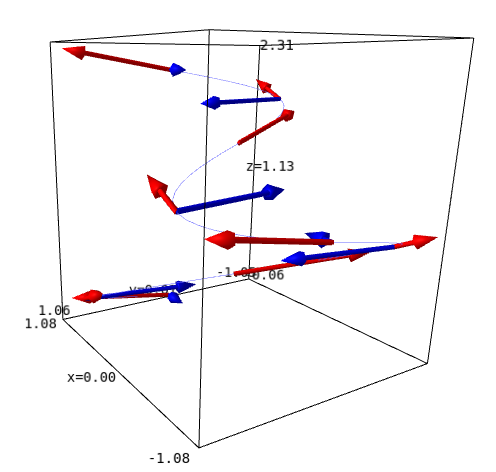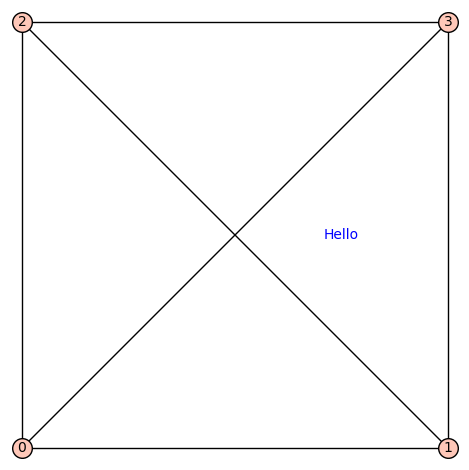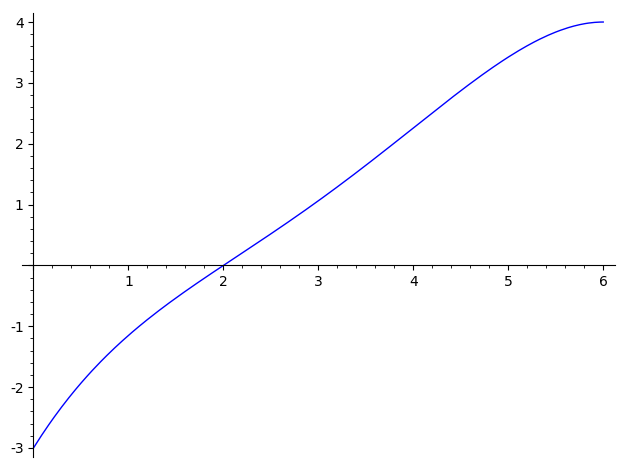2020-12-02 07:38:18 -0600 commented question What's the easiest way to calculate a Hironaka standard basis using SageMath? Is this related to local orderings as defined in Singular? 2020-12-01 17:58:10 -0600 answered a question Indexing variables in a list comprehension You can use R.gen(i-1), but it takes fewer keystrokes to slice the list of generators: sage: g = 3; n = 4 sage: R = PolynomialRing(QQ, ['lambda%s'%i for i in [1 .. g]] + ['psi%s'%i for i in [1 .. n]]) sage: lambdas = R.gens()[:g] sage: psis = R.gens()[n-1:] sage: lambdas, psis ((lambda1, lambda2, lambda3), (psi1, psi2, psi3, psi4))  Another trick is to start your indexing at 0 so that you can name your list like psi and then psi will refer to the variable named psi0. Or you can shift your list, or make a dictionary, if you want indices to start at 1. 2020-11-23 15:46:33 -0600 commented answer Klee-Minty cube vertices for D=5 Don't worry; an expert is someone who has made every possible mistake :) 2020-11-23 13:57:47 -0600 answered a question Klee-Minty cube vertices for D=5 In addition to the typo, you displayed the correct transpose(AA) but you accidentally passed the un-transposed AA as the ieqs argument to Polyhedron. It should be: sage: pol = Polyhedron(ieqs=transpose(AA)) sage: pol.Hrepresentation() (An inequality (-1, -4, -8, -16, -32) x + 3125 >= 0, An inequality (0, -1, -4, -8, -16) x + 625 >= 0, An inequality (0, 0, -1, -4, -8) x + 125 >= 0, An inequality (0, 0, 0, -1, -4) x + 25 >= 0, An inequality (0, 0, 0, 0, -1) x + 5 >= 0, An inequality (1, 0, 0, 0, 0) x + 0 >= 0, An inequality (0, 0, 0, 0, 1) x + 0 >= 0, An inequality (0, 0, 0, 1, 0) x + 0 >= 0, An inequality (0, 0, 1, 0, 0) x + 0 >= 0, An inequality (0, 1, 0, 0, 0) x + 0 >= 0) sage: len(pol.vertices()) 32 sage: pol.vertices() (A vertex at (3125, 0, 0, 0, 0), A vertex at (865, 505, 0, 5, 5), A vertex at (1625, 125, 125, 0, 0), A vertex at (1225, 325, 25, 25, 0), A vertex at (1385, 245, 65, 5, 5), A vertex at (1465, 205, 85, 0, 5), A vertex at (785, 545, 0, 0, 5), A vertex at (625, 625, 0, 0, 0), A vertex at (1025, 425, 0, 25, 0), A vertex at (2725, 0, 0, 25, 0), A vertex at (2885, 0, 0, 5, 5), A vertex at (2125, 0, 125, 0, 0), A vertex at (2525, 0, 25, 25, 0), A vertex at (2365, 0, 65, 5, 5), A vertex at (2285, 0, 85, 0, 5), A vertex at (2965, 0, 0, 0, 5), A vertex at (0, 0, 0, 0, 5), A vertex at (0, 0, 0, 0, 0), A vertex at (0, 505, 0, 5, 5), A vertex at (0, 125, 125, 0, 0), A vertex at (0, 325, 25, 25, 0), A vertex at (0, 245, 65, 5, 5), A vertex at (0, 205, 85, 0, 5), A vertex at (0, 545, 0, 0, 5), A vertex at (0, 625, 0, 0, 0), A vertex at (0, 425, 0, 25, 0), A vertex at (0, 0, 0, 25, 0), A vertex at (0, 0, 0, 5, 5), A vertex at (0, 0, 125, 0, 0), A vertex at (0, 0, 25, 25, 0), A vertex at (0, 0, 65, 5, 5), A vertex at (0, 0, 85, 0, 5))  2020-11-21 13:18:25 -0600 received badge ● Nice Answer (source) 2020-11-20 16:01:09 -0600 answered a question Plot velocity and acceleration vectors of a space curve The problem is that calling v3(k*pi/2) will try to call each component of v3 with that positional argument, but the last component of v3 is 1/6, so calling it with a positional argument is not supported. You can call it with a keyword argument instead, by using v3(t=k*pi/2). To be even more explicit, you can write v3.subs(t=k*pi/2). Similarly for a3. Or, what you probably meant to do: make v3 callable too: v3 = diff(r3,t) a3 = diff(v3,t)  With both approaches, your code works:2020-11-20 15:39:57 -0600 answered a question Error generating finite field The variable x in SageMath is pre-defined to be a symbolic variable. That is why the right-hand side of F1. = GF(2**8, modulus=x^8 + x^6 + x^5 + x^4 + x^3 + x + 1)  is valid. This assignment statement however re-defines x to be the generator of F1. To be precise, the assignment statement above is equivalent to: F1 = GF(2**8, modulus=x^8 + x^6 + x^5 + x^4 + x^3 + x + 1, name='x'); x = F1.gen()  Then, any future polynomial expression in x will be an element of F1; its normal form (which is automatically computed) will be written as a polynomial of degree less than 8. Hence, the right-hand side of the next statement F2. = GF(2**8, modulus=x^8 + x^4 + x^3 + x^2 + 1)  is not valid, because Sage will do its best to convert the given modulus to a polynomial over GF(2), and (because elements of F1 are written in normal form), the degree of that polynomial will be less than 8. Your second variant works because x is not overwritten. If you do not need a variable to hold the generator of your field, just write e.g.  F1 = GF(2**8, modulus=x^8 + x^6 + x^5 + x^4 + x^3 + x + 1, name='a')  as above. But in general, be careful about naming your variables and not accidentally overwriting them. Also, it is better to explicitly define e.g. x = polygen(GF(2)) rather than depending on Sage to have the symbolic x. 2020-11-19 04:19:50 -0600 received badge ● Nice Answer (source) 2020-11-18 17:53:29 -0600 commented answer How can I add arbitrary text to a Graph object You're welcome. You can accept an answer by clicking the ✅ 2020-11-18 15:49:23 -0600 answered a question How can I add arbitrary text to a Graph object To add text to a plot, literally add the result of a call to text to the graphics object returned by plot: sage: G = Graph([(0,1),(0,2),(0,3),(1,2),(1,3),(2,3)]) sage: G.plot(pos={ 0: (0,0), 1 : (1,0), 2: (0,1), 3: (1,1) },axes=False) + text("Hello",(0.75, 0.5))2020-11-11 16:22:46 -0600 received badge ● Nice Answer (source) 2020-11-11 10:44:13 -0600 commented question assignment vs. subs() The assignment t=I4 overwrites the variable t so that it no longer refers to a symbolic variable but rather to the concrete matrix I4, hence t^2 does give the squared matrix (and no symbolic variables are used in this computation). 2020-11-11 10:33:57 -0600 answered a question assignment vs. subs() There is no implementation for substituting a matrix into a symbolic expression, because the operation is not well-defined in general. (For example, what should happen when you substitute a matrix into exp(-1/t)?) Of course it is well-defined for polynomials. This substitution is implemented, but only for polynomials as members of a polynomial ring (rather than symbolic expressions), so you have to do a conversion: sage: t2.polynomial(QQ).subs(t=I4) [16 0 0 0 0] [ 0 16 0 0 0] [ 0 0 16 0 0] [ 0 0 0 16 0] [ 0 0 0 0 16]  It is easier (in life in general) to avoid symbolic expressions altogether, and to define t as a generator of a polynomial ring (instead of a symbolic variable), so that substitutions into polynomials in t work immediately: sage: t = polygen(QQ, name='t') sage: t^2 t^2 sage: (t^2).subs(t=I4) [16 0 0 0 0] [ 0 16 0 0 0] [ 0 0 16 0 0] [ 0 0 0 16 0] [ 0 0 0 0 16]  2020-11-10 15:46:49 -0600 commented question Braces inside a plot When I run the code, the brace is nowhere near the $x$ label. Where do you want the brace exactly? 2020-10-31 07:26:44 -0600 commented question All rational periodic points Probably it is a good idea to ask on MathOverflow. 2020-10-31 06:09:40 -0600 answered a question Tonelli-Shank values are incorrect when trying to get back to c value for Rabin It seems you have found two solutions $r_1,r_2$ to the equation $r^2 = c \pmod p$, instead of modulo $n=p^2$. These can be lifted to solutions of $r^2 = c \pmod n$ as explained in Tonelli's 1891 note: sage: x1 = r1.powermod(p, n) * c.powermod((n - 2*p + 1)/2, n) % n sage: x1 64703986196590532550677581867968606868573389071252692910980134129544137251401009133960328088692271753034649923142113830515792268064444518487016929096020442400942507965121543243161654445051643484581747767916266843209412116392813513581705574559159767553746511654597410103495145251789022071249050813249711591476 sage: (x1^2 - c) % n 0 sage: x2 = (-x1) % n sage: x2 89179848125512992787564462241620177844581389814477898537473698968367979714250257351636037110348977598736309799901497369527812510845450258136902578048921346119523079429281621431693965762644622048754271598699645 sage: (x2^2 - c) % n 0  2020-10-26 10:01:46 -0600 commented question Cohomology ring of a Lie algebra Could you add the code you have so far? 2020-10-21 05:26:31 -0600 answered a question Plot the solution of a system of equations sols = solve([f(0)==-3, f(2)==0, f2(4)==0, f1(6)==0, f(6)==4], a,b,c,d,e) plot(f.subs(sols),(x,0,6))2020-10-18 05:09:15 -0600 answered a question plot of sine, parabola intersection works but solve makes no sense It makes sense: it tried to solve symbolically, and it didn't get very far (it isolated the square, and took ± the square root). You don't want to solve symbolically but numerically. You want to find the two real zeros of x^2 - 5 - sin(x), and from the picture you (roughly) know intervals where these are located, so you can use find_root: sage: find_root(x^2 - 5 - sin(x), -5, 0) -2.025211637444818 sage: find_root(x^2 - 5 - sin(x), 0, 5) 2.3846766601465696  2020-10-17 19:16:40 -0600 commented question Problem with factor and imag. Weird. assume(z, 'complex') seems to help. 2020-10-17 17:37:53 -0600 answered a question Defining a function with different symbolic expressions in different parts of its domain specified by conditional statements If your goal is to plot, then you only need a function that accepts numbers and returns numbers: def f(x): if -2 <= x < -1: return x + 1 elif -1 <= x < 0: return 0 elif 0 <= x < 1: return x^2 elif 1 <= x <= 3: return x^3  Indeed, sage: plot(f,(-2,3))Now, this function does not play well with symbolic variables: sage: var('x') sage: print(f(x)) None  It is because inequalities with symbolic variables only evaluate to True when Sage can prove them; here none of them can be proved because nothing is known about x. If you add assumptions then you can achieve something: sage: assume(0<=x) sage: assume(x<1) sage: f(x) x^2  But it does not help with plotting. The above explains why e.g. var('x'); plot(f(x),(x,2,3)) does not work. You can have univariate piecewise-defined symbolic functions in Sage, to some extent: sage: var('x') sage: f = piecewise([([-2,-1], x+1), ((-1,0), 0), ((0,1), x^2), ([1,3], x^3)]) sage: plot(f,(-2,3))  It gives the same plot. Now it is also possible to evaluate f(x) for symbolic x: sage: f(x) piecewise(x|-->x + 1 on [-2, -1], x|-->0 on (-1, 0), x|-->x^2 on (0, 1), x|-->x^3 on [1, 3]; x) sage: plot(f(x),(x,-2,3))  Again, the same plot. This 'piecewise' functionality is unfortunately somewhat fragile, e.g. diff(f,x) returns junk. To plot a surface with a piecewise parametrization, avoid symbolic variables: def g(x,y): if x >= y: return x else: return y parametric_plot3d([lambda x,y: x, lambda x,y: y, g], (-1,1), (-1,1))  Your surface: x_3, y_3 = 1/3, 1/4 def X(u,v): return u def Y(u,v): return v def Z(u,v): if 1-u-v <= 0 or u <= 0 or v <= 0: # when P=(u,v) is outside of B or lies on its boundary return 0 else: # i.e., exactly when P lies in the interior of B return exp(-((u-x_3)^2+(v-y_3)^2)/((1-u-v)^2*(u^2)*(v^2))) print(Z(x_3,y_3)) parametric_plot3d([X,Y,Z], (x_3-0.1,x_3+0.1), (y_3-0.1,y_3+0.1), plot_points=[200,200], viewer='threejs') parametric_plot3d([X,Y,Z], (-2,2), (-2,2), plot_points=[200,200], viewer='threejs')  2020-10-16 09:36:23 -0600 answered a question 'builtin_function_or_method' object has no attribute 'split' Indeed if line = f.readline then line.split(delimiter) does not work, because in this case line is not a string but a method, so you cannot call split on it. You meant line = f.readline(). 2020-10-14 15:07:42 -0600 received badge ● Good Answer (source) 2020-10-14 13:37:12 -0600 received badge ● Nice Answer (source) 2020-10-14 11:06:58 -0600 answered a question Is there a reliable way to check if an object is a vector? You want is_Vector: sage: from sage.structure.element import is_Vector sage: v = vector([1,2,3]) sage: is_Vector(v) True sage: is_Vector([1,2,3]) False  It checks whether the input is an instance of the abstract element class Vector. 2020-10-10 07:07:10 -0600 commented answer Refinement between Lists of lists Nice one! :) 2020-10-07 13:36:14 -0600 commented question Multipartitions of a multiset in Sage Generate partitions of list(range(len(L))) and apply $k \mapsto L[k]$ to each element in each part? 2020-10-07 02:57:31 -0600 commented question Obtaining a simplicial complex associated to a poset with the help of Sage Please add the code you have so far, with an example $P$. 2020-10-03 10:23:22 -0600 commented answer Translating GAP-output into sage for lattices The different labeling seems to be due to FindStat, but they don't explain anywhere how their canonical labeling works, as far as I can see. 2020-10-03 10:02:12 -0600 received badge ● Nice Answer (source) 2020-10-03 09:53:59 -0600 answered a question Translating GAP-output into sage for lattices So essentially you want to turn a leq-matrix into a LatticePoset and get its Hasse diagram: sage: M = Matrix([ [ 1, 1, 1, 1, 1 ], [ 0, 1, 1, 1, 1 ], [ 0, 0, 1, 1, 1 ], [ 0, 0, 0, 1, 1 ], [ 0, 0, 0, 0, 1 ] ]) sage: L = LatticePoset((range(M.nrows()), lambda i,j: M[i,j] == 1)) sage: H = L._hasse_diagram sage: (H.edges(labels=False), len(H)) ([(0, 1), (1, 2), (2, 3), (3, 4)], 5)  I don't understand why you write a different labeling of the vertices, but I hope this helps. 2020-10-01 02:40:37 -0600 received badge ● Good Answer (source) 2020-10-01 01:45:14 -0600 received badge ● Nice Answer (source) 2020-09-30 16:00:54 -0600 answered a question Defining 4jm Wigner symbols With this syntax you are trying to create a callable symbolic expression. It doesn't work because the symbolic sum that you are using requires a symbolic expression (depending on the symbolic summation index m here) as the first argument, and wigner_3j in Sage does not accept symbolic arguments, only numeric arguments (more precisely, only integers or half-integers). This explains the errors. (By contrast, Sage does support symbolic binomial coefficients and sums, and hence callable symbolic expressions involving them.) In this case you have no need for symbolics at all; you can just define a plain Python function, using the plain (non-symbolic) sum: wigner_4j = lambda j1,j2,j3,j4,j,m1,m2,m3,m4: sum(wigner_3j(j1,j2,j,m1,m2,m)*wigner_3j(j,j3,j4,-m,m3,m4) for m in range(-j,j+1))  Or, without lambda: def wigner_4j(j1,j2,j3,j4,j,m1,m2,m3,m4): return sum(wigner_3j(j1,j2,j,m1,m2,m)*wigner_3j(j,j3,j4,-m,m3,m4) for m in range(-j,j+1))  2020-09-30 14:32:59 -0600 answered a question Evaluate a curl at a point? It is shown on the bottom of the reference manual page for vector fields, using the at method: p = E((0,0,0), name='p') show(curl_v.at(p).display())  $$\mathrm{curl}\left(v\right) = -2 e_{ z }$$ (For some reason, the point is not displayed in the notation. Not sure why this is. I would expect it as a subscript.) 2020-09-21 23:25:03 -0600 received badge ● Good Answer (source) 2020-09-21 10:09:55 -0600 received badge ● Nice Answer (source) 2020-09-19 09:28:26 -0600 received badge ● Nice Answer (source) 2020-09-19 07:00:05 -0600 received badge ● Nice Answer (source) 2020-09-19 06:38:19 -0600 answered a question How to express elements in a field of prime order and power of a prime order using the same function? This method is currently available only for the Givaro and NTL implementations of finite fields. You can specify the implementation using the impl keyword to GF, e.g.: sage: F. = GF(5^1, impl='givaro') sage: F.fetch_int(4) 4  In theory, this method could easily be added to all implementations. You might open a trac ticket for it and/or bring it up on the sage-devel mailing list.